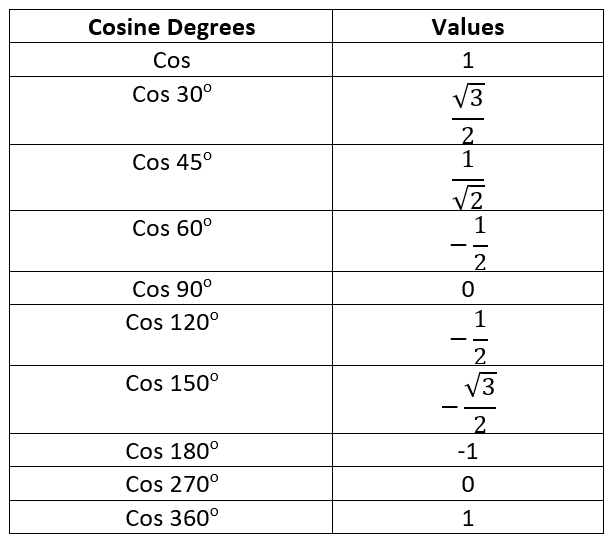# Cosine Worksheets

What is the Trigonometric Function Cosine? Notice right angle triangle. Angle's Cosine= length of the close to side A. Length(L) of A(side)/(L) of the hypotenuse(H). Draw triangle's each vertex and observes how you will estimate Cosine A & C. The Cosine function is the most usual trigonometric functions. In a right-angled triangle, angle Cosine = A(adjacent side) / L of H. Short form of Cosine is cos, and its formula will be: Cos x = (A)(H). Here always the CAH means: Cosine is adjoint(A) over hypotenuse(H). E.g., You have to find Cosine of triangle angle C, and you know Cosine = angle side of the triangle (A) and Cosine / (H). Then, you will assume the value that will be: (A) is the side of Length = 26, (H of Length) = 30. Now, the formula will be like this: Cos (C) = 26 / 30. Then, you will divide the values as mentioned above. You will get the answer that is 0.8666. Hence, The Cosine of 30° is equal to 0.8666. In a mathematical form: Cos 30 = 0.8666. Use calculator for Cosine of 30° and you must have 0.8666. Only make sure the calculator must be in degrees, not in radians.

• ### Basic Lesson

Guides students solving equations that involve an Cosines. Demonstrates answer checking.

• ### Intermediate Lesson

Demonstrates how to solve more difficult problems.

• ### Independent Practice 1

A really great activity for allowing students to understand the concept of Cosines.

• ### Independent Practice 2

Students find the Cosines in assorted problems. The answers can be found below.

• ### Homework Worksheet

Students are provided with problems to achieve the concepts of Cosines.

• ### Skill Quiz

This tests the students ability to evaluate Cosines.

Answers for math worksheets, quiz, homework, and lessons.

### What is the Importance of the Trigonometric Function Cosine?

Cosine is an important trigonometric function to calculate. Primarily because there are many functions in advanced mathematics and physics that use cos in their calculations. Thus, making it an important trigonometric function.One of the most interesting aspects of cos that its value changes according to the quadrants. You can observe the table above. Another important function to look at is that the graph of cos is similar to the sine graph. However, at the origin, the graph shows 90° , while the sine shows 0° .

#### W.S. Gilbert in the Pirates of Penzance

“I'm very good at integral and differential calculus, I know the scientific names of beings animalculous; in short, in matters vegetable, animal, and mineral, I am the very model of the modern Major General.”